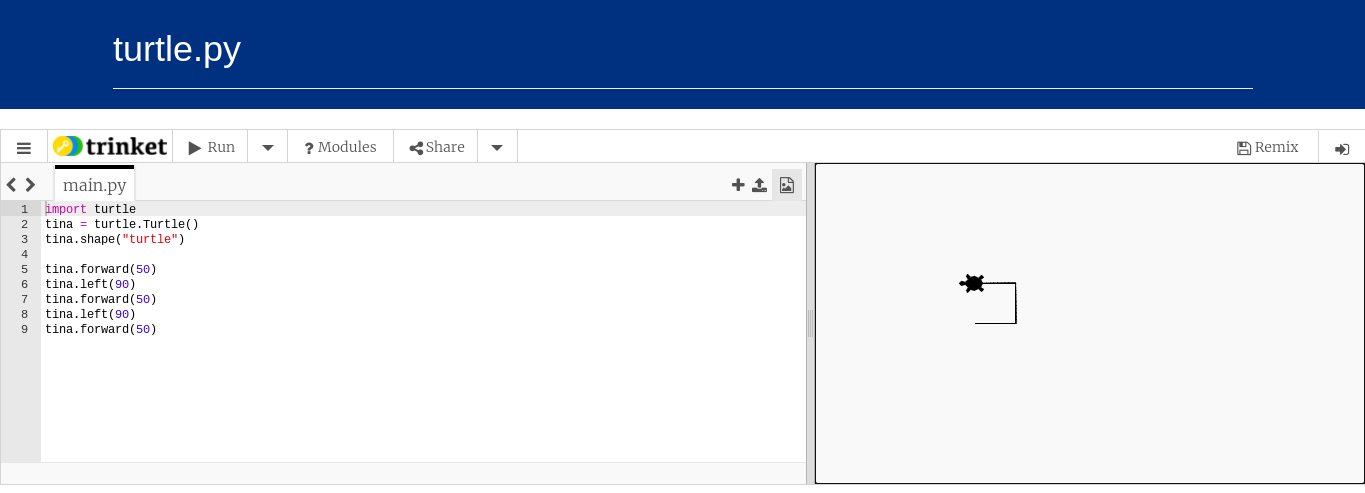Sept. 20, 2021Originally posted: Sept. 20, 2021
Last edited: 2 years, 2 months ago

# Variables in Python

In this lecture you will learn about variables, by the end of the lecture you will have good understanding the of following:

• How to declare a variable in Python
• Variables naming rules (conventions)
• How to assign a value to a variable

# What is a variable ?

variable simply is name (reference) to a memory location in the computer. A variable holds a value and this value may change. So you can imagine that a variable is a BOX that we can put a stuff in.

In reality, Python does not have variables. Instead it creates object references to refer to data objects. Data objects hold our data like numbers, words, group of values.

In the following lectures and throughout the course, when we say variable or object reference, we mean the same thing.

In this lecture we will talk about object references that refer to numbers (whole numbers without fractions).

# How to create an object reference in Python:

#### The syntax is simple:

``                       object reference (variable) = value``

```x = 10
```

If we type the above line of code in Python, then we have created an object of type int with a value 10 and an object reference x that refers to the int object.

Keep it simple, just say "x is referring to an int object with value 10"

To check the value of x, you can use the built-in print function and it should print 10.

Check out this example:

```x = 10
print(x)
```

`10`

The = operator in Python works as follows. The = operator binds an object reference to an object in memory.

There are two (2) cases here:

1. If the object reference does not exist, Python creates it by the = operator.
1. If the object reference already exists, it is simply re-bound to refer to the object on the right of = operator.

You can also change the value of x as follows:

```# case1
x = 10
print(x)

# case2
x = 20
print(x)
```
```10
20```

`In the next example we will introduce you to turtle.py. You can find this learning tool in the Resources tab:`
``

`All of the examples below assume you have already created a turtle named tina. Play around with the various commands in the list, experimenting with as many of them as you can. Try to make some cool designs! Get comfortable with typing code precisely and recovering from errors. Your work will be automatically saved.`

` `

Turtles can be all sorts of shapes, the coolest of which is turtle shape.

``tina.shape("turtle")``

`circle` and `arrow` are two others. We will learn later how to make our own.

Turtles are great for learning because you can treat them like little robot animals. Tell them to go forward or backward a certain number of pixels.

``````tina.forward(100)
tina.backward(100)``````

Now, a square:

``````tina.forward(100)
tina.left(90)
tina.forward(100)
tina.left(90)
tina.forward(100)
tina.left(90)
tina.forward(100)``````

The number passed to `left` and `right` should be a number of degrees. See what happens if you pass in a number bigger than 360.